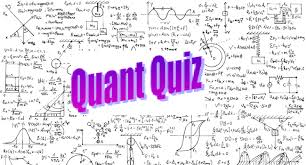## Quant Quiz for Railways, SSC CPO, SSC CGL 2016, SSC CHSL

###1.The difference between a discount of 40% on Rs 500 and two successive discount of 36%, 4% on the same amount is :
(a) Rs 0
(b) Rs 2
(c)Rs 1.93
(d)Rs 7.20

2.Rs.460 was divided among 41, beggars and children such that each beggarRs.12 and each children got Rs.8. What is the number of beggars?
(a) 33
(b) 30
(c) 36
(d) 28

3.A sum of money doubles itself at some rate of compound interest in 15 years in how many years will it become eight times of itself with the same rate ?
(a) 30
(b) 45
(c) 48
(d) 60

4.Find the compound interest on Rs. 15000 if rate of interest is 10%, 20% and 25% for three successive years compounded annually.
(a) Rs. 8700
(b) Rs. 9750
(c) Rs. 10500
(d) Rs. 12500

5.A man deposits Rs. 8000 in his bank account which pays interest at simple interest per annum. After 3 years, he deposits Rs. 6000 in his account. If after further 5 years, his closes his account after withdrawing his total account balance of Rs. 18700, then find the rate of interest.
(a) 5%
(b) 3%
(c) 2.5%
(d) 3.5%

6.75 weavers can complete a work in 30 days. 20 days later, they are joined by 50 more weavers but the efficiency of these new weavers is 50% of the efficiency of the existing weavers. In how many days less than the scheduled time will the work be completed?
(a) 2.5 days
(b) 5days
(c) 7.5 days
(d) 10 days

7.A 10liters mixture of milk and water contains 20% milk. 25% of the water contents are evaporated after boiling. Find the ratio of milk and water left in the mixture.
(a) 1:3
(b) 1:2
(c) 2:3
(d) 1:1

8.Two trains 137 m and 163 m in the length are running towards each other on the parallel line, one at the rate of 42 kmph and another at 48 kmph. In what time will they be clear of each other from the moment they meet ?
(a) 10 sec
(b) 12 sec
(c) 9 sec
(d) 11 sec

9.The average mark in mathematics for 5 students was found to be 50. Later it was discovered that in the case of one student the marks 48 were misread as 84. The correct average is :
(a) 40.2
(b) 40.8
(c) 42.8
(d) 48.2

10.The monthly incomes of two persons are in the ratio 2:3 and their monthly expenses are in the ratio 5:9. If each of them saves Rs 600 per month, then their monthly incomes are.
(a) Rs 1,500; Rs 2,250
(b) Rs 1,200; Rs 1,800
(c) Rs 1,600; Rs 2,400
(d) Rs 1, 4 00; Rs 2,100